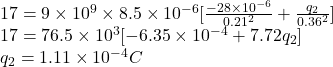## Two point charges are fixed on the y axis: a negative point charge q1 = -28 µC at y1 = +0.21 m and a positive point charge q2 at y2 = +0.36

Question

Two point charges are fixed on the y axis: a negative point charge q1 = -28 µC at y1 = +0.21 m and a positive point charge q2 at y2 = +0.36 m. A third point charge q = +8.5 µC is fixed at the origin. The net electrostatic force exerted on the charge q by the other two charges has a magnitude of 17 N and points in the +y direction. Determine the magnitude of q2.

in progress 0
2 months 2021-07-31T04:20:33+00:00 2 Answers 1 views 0

5.35 x 10^-5 C

Explanation:

q1 = – 28 micro Coulomb =- 28 x 10^-6 C

q2 = ?

q3 = 8.5 micro Coulomb = 8.5 x 10^-6 C

y1 = 0.21 m

y2 = 0.36 m

q3 is at origin.

Let F1 is the force between q1 and q3 and F2 is the force between q2 and q3.

By use of Coulomb’s law, the force between the two charges is given by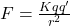Now,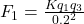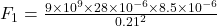F1 = 48.6 N    …. (1)

Now,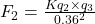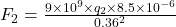F2 = 590277.78 q2   …. (2)

Now, F1 – F2 = 17

48.6 – 590277.78 q2 = 17     from (1) and (2)

q2 = 5.35 x 10^-5 C

Thus, the charge on q2 is 5.35 x 10^-5 C.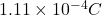Explanation:

Coulomb’s force: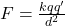where, q and q’ are two charges, d is the distance between them and k is the Coulomb constant.

The net force on charge q at origin is 17 N.